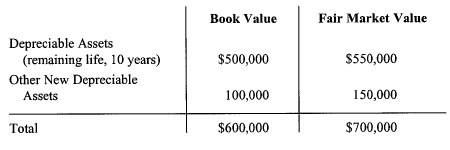Connect with us

# Recording Investment [Equity Method Vs. Fair Value Method]Published

onFirms buy bonds and stocks to generate investment revenues and dividend revenues as well as for speculative reasons. The different objectives for these investments dictate different accounting treatments to insure a fair reporting. Accordingly, this post examines the conventional accounting treatments and methods for investments in equity securities using equity method versus fair value method.

Under the equity method, the investment in common stock is initially recorded at cost, then is increased [decreased] by the investor’s share of investee income (loss) and decreased by all dividends received from the investee.

In other words:

Investment = Acquisition Cost + Investor’s Share of Investee Income – Dividends Received

Where:

Investor’s Share of Investee Income = (Investee’s Net Income x Percentage of Ownership) – Adjustments

and:

Dividends Received = (Total Dividends Paid by the Investee x Percentage of Ownership)

To illustrate the differences between the equity method and the fair value method, let’s assume the following example.

(1). On November 2, 2009, the LIE DHARMA Company acquired 5,000 shares (30% of the PUTRA Company common stock) at a cost of \$20 a share.

A. Under the fair value method (assuming no significant influence). The following entry would be made:

[Debit]. Available-for-Sale Securities = 100,000
[Credit]. Cash 100,000

B. Under the equity method (assuming significant influence). The following entry would be made:

[Debit]. Investment in PUTRA Stock = 100,000
[Credit]. Cash = 100,000

(2). In 2009 the LIE DHARMA Company reported a net income of \$100,000

A. Under the fair value method: No entry is required

B. Under the equity method:

[Debit]. Investment in PUTRA Stock (\$100,000 x 30%) = 30,000
[Credit]. Revenue from Investment = 30,000

(3). At the end of the year 2009, the fair value of PUTRA stock was \$30 per share

A. Under the equity method: No entry is required

B. Under the fair value method:

[Debit]. Securities Fair Value Adjustment [(\$30 – \$20)] x 5,000 = 50,000
[Credit]. Unrealized Holdings Gain or Loss-Equity = 50,000

(4). On February 1, 2010, the PUTRA Company paid a total cash dividend of \$50,000

A. Under the fair value method:

[Debit]. Cash = 15,000
[Credit]. Dividend Revenue (\$50,000 x 30%) = 15,000

B. Under the equity method:

[Debit]. Cash = 15,000
[Credit]. Investment in PUTRA Stock = 15,000

(5). In 2010, the PUTRA Company reported a \$100,000 loss

A. Under the fair value method: No entry is required

B. Under the equity method:

[Debit]. Loss on Investment = 30,000
Investment in PUTRA Stock (\$100,000 x 30%) = 30,000

(6). At the end of 2010, the fair value of the PUTRA stock was \$15 per share

A. Under the equity method: No entry is required

B. Under the fair value method:

[Debit]. Unrealized Holdings Gain or Loss Equity (\$20 – \$15) = 25,000
[Credit]. Securities Fair Value Adjustment = 25,000

Under the equity method, there is a need not only to periodically change the value of the investment account for the increases and decreases in the investor’s proportionate share of income and decreases for dividends received, but also the need to amortize the difference between the investor’s initial acquisition cost and the investor’s proportional share of the underlying book value of the investee at the date of purchase.

To illustrate, assume that the Lie Company purchases, on January 1, 2009, 30% of the 100,000 shares of the Dharma Company for \$200,000. On the date of acquisition the following information of the Dharma Company was available:Liabilities               = \$100,000
Common Stock      = \$250,000
Retained Earnings  = \$250,000
Total                      = \$600,000

The Dharma Company paid, on June 15, 2009, a cash dividend of \$30,000 and reported net income of \$90,000 and an extraordinary gain of \$20,000. The Lie Company will make the following entries:

(1). To record the purchase of 30,000 shares for \$200,000 on January 1, 2009

[Debit]. Investment in Dharma Stock = \$200,000
[Credit]. Cash = 200,000

(2). To record the dividend received of \$9,000 (\$30,000   30%) on June 15, 2009

[Debit]. Cash = 9,000
[Credit]. Investment in Dharma Stock = 9,000
(3). To record share of Dharma Company’s ordinary and extraordinary income, on December 31, 2009

[Debit]. Investment in Dharma Stock = 33,000
[Credit]. Revenue from Investment (ordinary) (\$90,000 x 30%) = 27,000
[Credit]. Gain from Investment (extraordinary) (\$20,000 x 30%) = 6,000
(4). To depreciate the proportionate share of any difference between the fair market and book value of investee depreciable assets

[Debit]. Revenue from Investment (ordinary) = \$1,500
[Credit]. Investment in Dharma Stock = 1,500

The excess of fair market value over book value of assets at the time of acquisition was \$50,000 =(\$550,000 -\$500,000). The Lie Company’s share is \$15,000 = (\$50,000 x 30%). The share per year is \$1,500 = (15,000/10).

(5). To depreciate goodwill

[Debit]. Revenue from Investment (ordinary) = \$750
[Credit]. Investment in Monti Stock = \$750
The goodwill at the time of acquisition is computed as follows:

1. Purchase Price = \$200,000

2. Book Value of Net Asset Acquired [30% x (\$600,000 – \$100,000)] = \$150,000

• Increase in depreciable assets [30% (\$550,000 – \$500,000)] = 15,000
• Increase in other depreciable assets [30% (\$150,000 – \$100,000)] = 15,000

4. Fair Market Value of Assets Acquired = (180,000)

5. Purchased Goodwill = 20,000

Assuming goodwill is amortized over 20 years, the yearly change is \$750 = \$15,000/20

Are you looking for easy accounting tutorial? Established since 2007, Accounting-Financial-Tax.com hosts more than 1300 articles (still growing), and has helped millions accounting student, teacher, junior accountants and small business owners, worldwide.